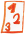Math worksheetsCustom math worksheets at your fingertips

Details for problem "Product of two sums term expansion"

Quickname: 1595

Summary

Expand a product term which has sums with variables as factors.

ExampleDescription

A product term whose factors are a product or number and a sum has to be expanded. The term may contain up to three variables.

The shape of the term to be expanded may be one of these:

a) (a+b)(c+d)
b) (a+b)(c-d)
c) (a-b)(c+d)
d) (a-b)(c-d)

The term can be chosen to be

- one of group a, which means both factors are sum terms.
- one of groups a,b or c, which means at most one factor is a difference term.
- any of gropups a-d, which means both factors can be difference terms
- one of groups b,c or d, which means at least one factor is a difference term.

Further,
- squares can be disallowed, which means a variable will appear only in one of the factor terms.
- it can be specified that one of the variables in each factor may be a number.

The range for the numbers can be chosen.

The number of problems is selectable. The first problem can be presented with the answer in the form of a sample problem. If required, some space will be left so that the answer may be written on the sheet.

Free worksheets with solution sheets for download

Download free worksheets for this math problem here. There is a second sheet with the solutions. Just click on the respective link.Worksheet 1Solution sheetWorksheet 2Solution sheetWorksheet 3Solution sheet

If you can not see the solution sheets for download, they may be filtered out by an ad blocker that you may have installed. If this is the case, please allow ads for this page and reload the page. The solution sheets will then reappear.

• Do the sample worksheets do not really fit?
• Do you need more math worksheets, with a different level of difficulty?
• Would you like to combine different problems on a worksheet and adjust them to your needs?
• As a teacher, you can put together your own worksheets using the automatically generated math problems provided.
With a free initial credit, you can start creating your own math worksheets in a few minutes.

It does not cost anything to try! Register here, to create custom worksheets now!

Customization options for this problem

ParameterPossible values
Number of problems1, 2, 3, 4, 5, 6, 7, 8, 9, 10
Number Range20, 50, 99, 200, 499, 999, 1999, 4999
Problem type(a+b)(c+d), (a-b) allowed, exactly one of (a-b), at least one (a-b)
Sample problemYes, No
Space for answerYes, No
Allow squaresYes, No
Numbers allowedYes, No

Similar problems

RemarkDescriptionName and direct link
expand product of product and sum termExpand product term with variables.Product term expansion

Deutsche Version dieser Aufgabe
These informational pages with samples describe math problems that can be combined on custom math worksheets with solutions for home and school use.
Please visit the dw math main page for more information!